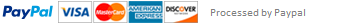### Implement a simple amortization utility with Python

Nothing too fancy, but you must meet the following design requirements. Imagine how these requirements might be made more realistic as you go through the exercise.

Naming

It must import "quietly" (no output on import statement) as:

import quiz5_your6+2 as quiz5

To be clear: write a module and save it to quiz5_your6+2.py

I.e., I would submit mine as quiz5_fergumk.py

Functionality

Simple contract representation

The module must implement a Terms class that can be instantiated by a dollar amount, a number of years (length of contract), number of payments per year (frequency), and an annual interest rate. I.e.:

my_terms = Terms(principal=1000, years=10, frequency=4, rate=4.0)

For one bonus point

The years and frequency parameters should be integers, and trying to use a float for either should raise a TypeError exception.

The Terms class will implement instance attributes:

principal - the dollar amount of the contract

fraction - the fraction (as a number between 0 and 1) representing the year fraction associated with a single payment

coupon_rate - the fraction (as a number between 0 and 1) representing the portion of the annual rate for a single payment

payments - the total number of payments in the contract In this example, this would mean the following would evaluate as True:

my_terms.principal == 1000

my_terms.fraction == 0.25

my_terms.coupon_rate == 0.01

my_terms.payments == 40

Numerical representation of the amortization schedule for that contract

The module must implement a method called amortization that takes a Terms object and returns a DataFrame with the following structure.

Index: 'Period' -- integer, human based (i.e. first period is 1, not 0)

Column names: 'Payment', 'Interest', 'Principal', and 'Outstanding'

Each row (Period) will be the amount paid, the amount of interest paid, the amount of principal paid, and the outstanding principal after the payment is made. The last row should have outstanding "close" to zero (rounding errors will creep in in most cases).

For two bonus points

Make all amounts round to pennies and make the final outstanding principal exactly zero; adjust the final payment, principal and interest amounts to make everything add up properly. You can only earn both of these -- no partial credit on bonus points.

Graphical representation of the amortization schedule

The module must implement a method called show_schedule that takes an amortization DataFrame as returned by the amortization method and shows two graphs, side by side: a stacked bar graph of interest and principal, and then a simple bar graph of the outstanding balance.

Bonus points

Full bonus points will only be awarded for submissions that are completely correct on the initial submission. If resubmitted for any reason, only half bonus points will be awarded.

This assignment has been answered 5 times in private sessions.